# H2 Math Sat 330pmThis page contains all questions and answers asked by students/ discussions from this class. The most recent questions will be at the top.

MF26

Recall the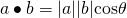If the angle is obtuse, then.
Thus,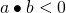Vectors Q7 [Homework] (i)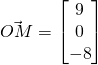Using ratio theorem,Sinceis perpendicular to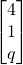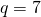(ii)
To be a parallelogram,(iii)
LetSince— (1)— (2)
Solving,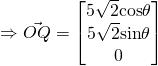Vectors Q9 [Homework] (i)Normal of(ii)
Letbe the acute angle(iii)— (1)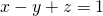— (2)

Using GC,(iv)
Let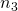be the normal ofLength of projection(v)
Required distance(vi)
Let normal ofIfintersect at l,lies onNot readable? Change text.